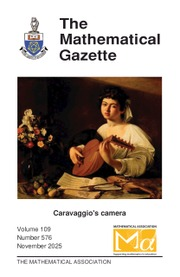Home
Hostname: page-component-684899dbb8-nlvjk Total loading time: 0.744 Render date: 2022-05-17T22:22:10.206Z Has data issue: true Feature Flags: { "shouldUseShareProductTool": true, "shouldUseHypothesis": true, "isUnsiloEnabled": true, "useRatesEcommerce": false, "useNewApi": true }The Mathematical Gazette

# The convexity of the function y = E(x) defined by xy = yx

Published online by Cambridge University Press:  02 March 2020

## Extract

The set of solutions to the equation xy = yx has been studied extensively over the past three centuries, including work by well known mathematicians such as Daniel Bernoulli (1700–1782), Leonhard Euler (1707–1783), and Christian Goldbach (1690–1764). Various mathematicians have focused on the integer, rational, real, and complex solutions. For example, it has been shown (see ) that the equality 24 = 42 gives the only distinct integer solutions. Our exposition below presents some of the key ideas behind the positive real solutions to this equation and illustrates how rational solutions can be found. To learn more about the various solutions, the reader can consult the articles listed at the end of this paper, as well as the extensive references given in these articles. It is also possible to find some of this material on the Web.

Type
Articles
Information
The Mathematical Gazette , March 2020 , pp. 36 - 43

## Access options

Get access to the full version of this content by using one of the access options below. (Log in options will check for institutional or personal access. Content may require purchase if you do not have access.)

## References

Gerrish, F., a b = b a: the positive integer solution, Math. Gaz. 76 (November 1992) p. 403.CrossRefGoogle Scholar
Cundy, H. M., x y = y x: an investigation, Math. Gaz. 71 (June 1987) pp. 131135.CrossRefGoogle Scholar
Siu, M., An interesting exponential equation, Math. Gaz. 60 (October 1976) pp. 213215.CrossRefGoogle Scholar
Sved, M., On the rational solutions of x y = y x, Math. Mag. 63 (1990) pp. 3033.CrossRefGoogle Scholar
Beardon, A., The real solutions of x y = y x, Math. Mag. 39 (1966) pp. 108111.Google Scholar
Lóczi, L., Two centuries of the equations of commutativity and associativity of exponentiation, Teaching Mathematics and Computer Science, 1-2 (2003) pp. 219233.CrossRefGoogle Scholar
Bennet, A. and Reznick, B., Positive rational solutions to x y = y mx; a number-theoretic excursion, Amer. Math. Monthly 111 (2004) pp. 1321.Google Scholar
Knoebel, R., Exponentials reiterated, Amer. Math. Monthly 88 (1981) pp. 235252.CrossRefGoogle Scholar

# Save article to Kindle

Note you can select to save to either the @free.kindle.com or @kindle.com variations. ‘@free.kindle.com’ emails are free but can only be saved to your device when it is connected to wi-fi. ‘@kindle.com’ emails can be delivered even when you are not connected to wi-fi, but note that service fees apply.

Find out more about the Kindle Personal Document Service.

The convexity of the function y = E(x) defined by xy = yx
Available formats
×

# Save article to Dropbox

To save this article to your Dropbox account, please select one or more formats and confirm that you agree to abide by our usage policies. If this is the first time you used this feature, you will be asked to authorise Cambridge Core to connect with your Dropbox account. Find out more about saving content to Dropbox.

The convexity of the function y = E(x) defined by xy = yx
Available formats
×

# Save article to Google Drive

To save this article to your Google Drive account, please select one or more formats and confirm that you agree to abide by our usage policies. If this is the first time you used this feature, you will be asked to authorise Cambridge Core to connect with your Google Drive account. Find out more about saving content to Google Drive.

The convexity of the function y = E(x) defined by xy = yx
Available formats
×
×